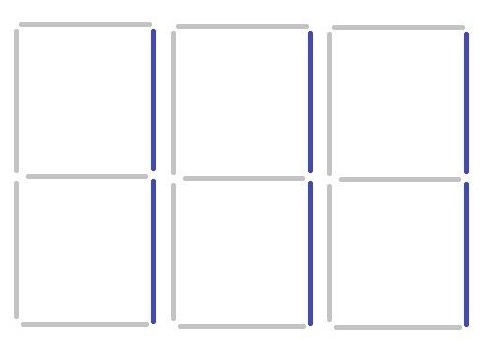# Maximum number that can be display on Seven Segment Display using N segments in C++

C++Server Side ProgrammingProgramming

Given the task is to find the maximum number that can be displayed using N segment on ant number of seven segment display.

Let’s now understand what we have to do using an example −

Input − N=5

Output − 71

Explanation − The largest number will be displayed as follows on the seven segment display −Input − N=6

Output − 111## Approach used in the below program as follows

• The following situation can be divided into 3 case −

• Case 1

If N is 0 or 1, then it is not possible to display any number.

• Case 2

If N is odd. Then the numbers that can be displayed with odd number of segments are 2, 3, 5, 7 and 8 out of which 7 takes the least number of segments, that is, 3. Therefore 7 is the most significant digit in this case.

• Case 3

If N is even. Then the numbers that can be displayed with odd number of segments are 0, 1, 4, 6, 9 and 8 out of which 1 takes the least number of segments, that is, 2. Therefore 1 is the most significant digit in this case.

• In function MaxNumber() first check for the base condition using

if (N == 1 || N == 0)

• Then using another if statement, check if N is even. If so then print “1” as it is the most significant digit in the even case and call MaxNumber(N - 2) as 2 segments are being used here.

• Use another if statement to check if N is odd. If so then print “7” as it is the most significant digit in the odd case and call MaxNumber(N - 3) as 3 segments are being used here.

## Example

Live Demo

#include <iostream>
using namespace std;
void MaxNumber(int N){
//Condition to check base case
if (N == 1 || N == 0){
return;
}
//If the number is even
if (N % 2 == 0){
cout << "1";
MaxNumber(N - 2);
}
//If the number is odd
else if (N % 2 == 1){
cout << "7";
MaxNumber(N - 3);
}
}
//Main function
int main(){
int N;
N = 5;
MaxNumber(N);
return 0;
}

## Output

If we run the above code we will get the following output −

71